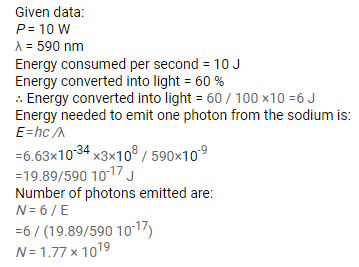# Calculate the number of photons emitted per second by

Question:

Calculate the number of photons emitted per second by a $10 \mathrm{~W}$ sodium vapour lamp. Assume that $60 \%$ of the consumed energy is converted into light. Wavelength of sodium light $=590 \mathrm{~nm}$

Solution: